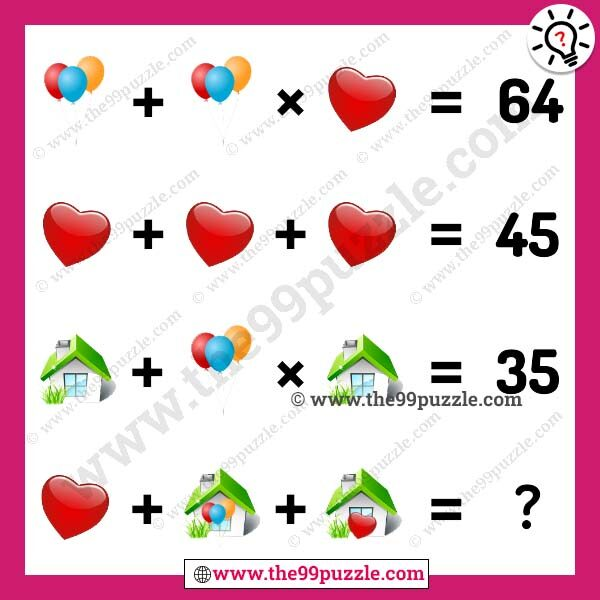# Balloon, love, home picture math equation – Puzz277

Can you solve this balloon, love, home picture math equation? In this puzzle picture, you see the three different pictures in the math puzzle equation. Genius students fail to solve this brain teaser puzzle. This math picture is more tricky than other picture puzzles. If you practice more math equation puzzles, find this website and try to solve all these puzzles. To learn and solve quickly math tricks by the practice of these puzzles math.###### Explanation:

Balloon=4

Love=15

Home=7

4+4×15 = 64

15+15+15 = 45

7+4×7 = 35

15+(7+4)+(7+15) = 48